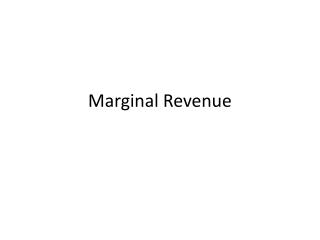# Marginal Revenue - PowerPoint PPT PresentationDownload PresentationMarginal Revenue

Marginal RevenueDownload Presentation## Marginal Revenue

- - - - - - - - - - - - - - - - - - - - - - - - - - - E N D - - - - - - - - - - - - - - - - - - - - - - - - - - -
##### Presentation Transcript

1. Marginal Revenue

2. Revenue is simply the amount of money a firm receives. If a firm is selling one product at a homogenous price (each unit sold is the same price) then total revenue will equal price times quantity(PxQ). • Marginal revenue is the increase in revenue from selling one more unit of a product. • For competitive firms, marginal revenue isn't very interesting. If all units are sold for the market price, then marginal revenue will simply be the market price. • However, marginal revenue is very different for monopolies. • It is computed by taking the difference between total revenues before and after an increase in the rate of production. If product price is constant, marginal revenue is the same as price. As long as the price of a product is constant, price and marginal revenue are the same, but it is not uncommon for additional output to be sold at a lower or higher price. • It plays a key role in the profit-maximizing decision of a monopoly • A monopoly maximizes profit by equating marginal revenue (the extra revenue generated from production), with marginal cost, (the extra cost of production). If these two marginals are not equal, then profit can be increased by producing more or less output.

3. The table on the next slide summarizes the marginal revenue received by a hypothetical firm, First Pharmaceutical. This firm owns the patent to Athsmabegone, the only cure for the ailment known as athsma. As the only producer of Athsmabegone, First Pharmaceutical is a monopoly with extensive market control, facing a negatively-sloped demand curve. To sell a larger quantity of Athsmabegone, First Pharmaceutical must lower the price.

4. The first column is the quantity of Athsmabegone sold, ranging from 0 to 12 ounces. The second column is the price First Pharmaceutical receives for selling this medicine, which ranges from \$4.50 to \$10.50 per ounce. The third column is the total revenue First Pharmaceutical receives for producing and selling this medicine.

5. One obvious point is that marginal revenue decreases with the quantity of medicine produced. A second point is that marginal revenue is less than the price of Athsmabegone for each quantity sold. The price of the fifth ounce of Athsmabegone is \$8, but the marginal revenue generated by the fifth ounce is only \$6. Marginal revenue is less than price. And because price is average revenue, marginal revenue is also less than average revenue.

6. Consider the situation facing the First Pharmaceutical monopoly. If Feet-First Pharmaceutical sets the Athsmabegone price at \$8.50, then buyers are willing to purchase 4 ounces. However, if First Pharmaceutical wants to increase the quantity sold from 4 ounces to 5 ounces, then it MUST lower the price from \$8.50 to \$8 per ounce. But here is the catch: First Pharmaceutical must lower the price for ALL, not just the extra ounce. • What happens to First Pharmaceutical's total revenue when it lowers the price? Two forces are at work: (1) the revenue gained by adding extra ounces and (2) the revenue lost by lowering the price for existing ounces. Marginal revenue is the net result of both.

7. First, by lowering its price, First Pharmaceutical increases the quantity sold from 4 ounces to 5 ounces. This extra ounce generates an extra \$8 of revenue, the price of the sixth ounce. This is \$8 of extra revenue that First Pharmaceutical did NOT have at the higher price. If this is all that happens, then First Pharmaceutical has a marginal revenue of \$8 for the sixth ounce, equal to the price. • Second, by lowering its price, First Pharmaceutical collects less revenue from its other 4 ounces. It would have collected \$8.50 per ounce for a total of \$34. But with the lower \$8 price it collects only \$32, a reduction of total revenue by \$2, or \$0.50 per ounce. • The \$8 gained by selling the extra ounce is partially offset by the \$2 lost from lowering the price for other ounces. On net, total revenue increases by only \$6. The loss of revenue collected on existing ounces is the key reason that marginal revenue is less than price.